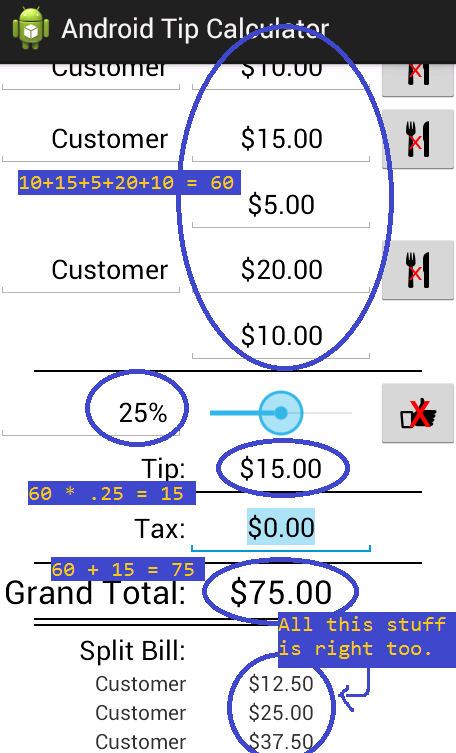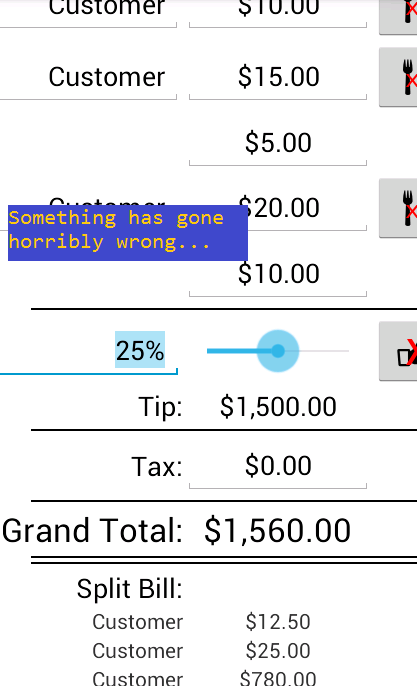glaukon
Chapter 3: Java - Part 20
We still need the TextView for the tip to work, so that's the next goal. We want it to say the total tip of all the Diners combined, or the total tip based on the grand total. Thus, while we're at it, we're also going to implement the code for the grand total calculation. Declare these variables:

private double grandTotal;

private double totalTip;

private TextView textGTotal;

private TextView tipDollar;

We'll use doubles to hold the value of the grand total and total tip amount, and we need access to the TextView for the grand total and the tip. Let's assign values next, in onCreate.

grandTotal = 0.0;

totalTip = 0.0;

textGTotal = (TextView) findViewById(R.id.textGTotal);

tipDollar = (TextView) findViewById(R.id.tipDollar);

We'll have grandTotal and totalTip start off at 0. As always, with the UI elements, I chose variable names that match the Ids. Now, let's create a new method in MainActivity called calcGrandTotal. We'll use this method whenever we change something that would affect the grand total amount, like when the user changes the tip, or edits an order EditText.

public void calcGrandTotal() {

grandTotal = 0.0;

for (int i = 0; i < dinerList.size(); i++) {

grandTotal += dinerList.get(i).total;

}

totalTip = grandTotal * tipPercentValue;

tipDollar.setText("\$" + String.format("%,.2f", totalTip));

textGTotal.setText("\$" + String.format("%,.2f", grandTotal + totalTip));

}

Let's run through this. Every time we want to calculate grandTotal, the first thing we do is reset it to 0. Then, we use a for loop to run through all the Diners in dinerList. For each Diner, we add its total value to grandTotal. I don't think you've seen this before, but you can access any object's public attributes by putting a dot after the object, and then the attribute's name. So to get a Diner's total attribute, we use Diner.total. It's similar to using an object's method, so make sure you don't get confused. You know we're accessing an attribute and not a method because there are no parentheses after the word total. Again, for those of you who are more experienced at programming, we're going to go over encapsulation in the next tutorial, and just ignore it in this one.
After the for loop, we calculate totalTip's value by multiplying grandTotal by tipPercentValue. Then we set tipDollar's Text to totalTip (after formatting), and set textGTotal's Text to grandTotal plus totalTip (after formatting). We now have a method that calculates the grand total, uses it to get the total tip, and then sets the corresponding TextViews accordingly. Of course, since we haven't called it anywhere in our code, this method doesn't actually get to do anything yet. We need to call calcGrandTotal every time we want to update the tip/grand total. Thus, add it to the onProgressChanged method, as the last line of the if block, like so:

public void onProgressChanged(SeekBar seekBar, int progress, boolean fromUser) {

if (seekBar == tipSlider) {

...

calcGrandTotal();

}

}

Now, after doing all the tip calculations in onProgressChanged, we'll execute the grand total calculations. Next, go to the onFocusChange method and add the same line. This time, put it inside the if block that calls updateTotal. Make sure you call calcGrandTotal after the updateTotal, like so:

public void onFocusChange(View v, boolean hasFocus) {

...

if (v == dinerList.get(i).orderList.get(j) && hasFocus == false) {

dinerList.get(i).updateTotal((EditText) v, tipPercentValue);

calcGrandTotal();

}

Now, every time the user changes an order EditText, we update the total for the Diner that order belongs to, and then we update the grand total, taking into account the Diner's new total value. Test it out in an AVD. Use a bunch of Diners and a bunch of orders. Then slide tipSlider around a bit. You should see everything updating in real-time, including the TextViews for the total tip and grand total. The math should also be correct, although you're very welcome to verify it yourself.Okay, let's not get too excited. There's actually a bug here. Let's squash it. First, click on tipPercent (the EditText for the tip). Now, without changing it, click on any order EditText. Again, without changing it, click out of the EditText. See the problem?In the above screenshot, it would appear as if our app has multiplied the pre-tip total, \$60.00, by 25, rather than 25%. Thus, we have a rather unreasonable total tip of \$1,500.00. Fortunately, this is another great opportunity to learn some debugging tricks.